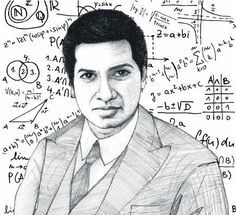# Django full text search (Postgres)

Let's say we have a large database, few milions row, one-to-many relationship models with some text field, and we want to search some keywords.

Traditional way will be real pain and slow, I know. So let's do something smart and enjoy lightning-fast execution with Full Text Search.Suppose we have an app named main and it's models look like this

from django.db import models

class Parent(models.Model):
title = models.CharField(max_length=255)

class Child(models.Model):
parent = models.ForeignKey(Parent, on_delete=models.CASCADE, related_name="children")
content = models.TextField(null=True, blank=True)


For example, we want to search keyword in childs content, and we want to do it quick! You can do like this, but that not quick enough for me. Maybe because I have too damn much data. But there are other way. First we need to add this line to settings.py

settings = [
# ...
"django.contrib.postgres",
# ...
]


Then we add SearchVectorField to the model

search_vector = SearchVectorField(null=True, blank=True)


Finally we can create index

from django.contrib.postgres.search import SearchVectorField
from django.contrib.postgres.indexes import GinIndex

class Child(models.Model):
content = models.TextField(null=True, blank=True)
search_vector = SearchVectorField(null=True, blank=True)

class …

# My math notes - Part 1

## Sequence

https://en.wikipedia.org/wiki/Sequence

• Sequence is like list
• Often write like this: $a_n, b_n, c_n$

Example: $\{(p_t, v_t)_{t=1,...,T}\}$ where $p_t$ is the price associated with tick t and $v_t$ is the volume associated with tick t. The so-called tick rule defines a sequence $\{b_t\}_{t=1,...,T}$ where

$b_t= \begin{cases} b_{t-1} &\text{if $$\Delta{p_t}=0$$} \\ \frac{\Delta{p_t}}{\Delta{p_t}} &\text{if $$\Delta{p_t}\neq0$$} \end{cases}$

# Đứa con của Thượng đế - Ramanujan## I. Thuở sơ khai

Ngày xưa mình đọc được trong những quyển sách, bài báo, rằng có những người đạt được những năng lực tưởng như siêu nhiên, kiểu như thần đồng toán học không cần ai chỉ dạy cũng có thể hiểu và làm được những phép toán kỳ diệu.

Mình thì mình không tin đâu.

Page 1 / 2 »